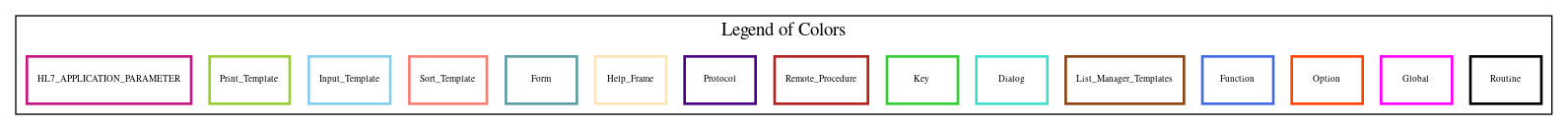Home   Package List   Routine Alphabetical List   Global Alphabetical List   FileMan Files List   FileMan Sub-Files List   Package Component Lists   Package-Namespace Mapping
Routine: SDWLE111

Routine: SDWLE111

Information

SDWLE111 ;;IOFO BAY PINES/TEH - WAITING LIST-ENTER/EDIT - WAIT LIST TYPE/INSTUTITION;20 Aug 2002

Source Information

Source file <SDWLE111.m>

Call GraphCall Graph Total: 5

Package Total Call Graph
VA FileMan 4 ^DIC  ^DIE  \$\$EXTERNAL^DILFD  ^DIR
Scheduling 1 ^SDWLSC

Caller Graph

Legends:Package Component Superscript legend

 action A extended action Ea event driver Ed subscriber Su protocol O limited protocol LP run routine RR broker B edit E server Se print P screenman SM inquire ICaller Graph Total: 1

Package Total Caller Graph
Scheduling 1 SDWLE

Entry Points

EN ;
EN0 ;
EN1
EN9
EN10 ;SERVICE CONNECTION - SD*5.3*394
END
GETTM ;CHECK WAIT LIST FOR TEAM ASSIGNMENT.

External References

Name Field # of Occurrence
^DIC EN10+19
^DIE EN9+5, EN10+24
\$\$EXTERNAL^DILFD EN+5, EN10+11
^DIR EN9
^SDWLSC EN10+2

Interaction Calls

Name Line Occurrences
Function Call: WRITE
• Prompt: !,"Wait List Type: ",\$\$EXTERNAL^DILFD(409.3,4,,SDWLTY)
• Line Location: EN+5
Function Call: WRITE
• Prompt: "// (No Editing)"
• Line Location: EN+5
Function Call: WRITE
• Prompt: " Required or '^' to Quit"
• Line Location: EN9+0
Function Call: WRITE
• Prompt: " Invalid Entry."
• Line Location: EN9+4
Function Call: WRITE
• Prompt: !
• Line Location: EN10+9
Function Call: WRITE
• Prompt: "Required or '^' to Quit."
• Line Location: EN10+19
Routine Call
• DIC
• Line Location:
• EN10+19
Routine Call
• DIE
• Line Location:
• EN9+5
• EN10+24
Routine Call
• DIR
• Line Location:
• EN9

FileMan Files Accessed Via FileMan Db Call

FileNo Call Tags
^SDWL(409.3 - [#409.3] Classic Fileman Calls,  EXTERNAL^DILFD
^SDWL(409.32 - [#409.32] EXTERNAL^DILFD

Global Variables Directly Accessed

Name Line Occurrences  (* Changed,  ! Killed)
^SCTM(404.51 - [#404.51] EN10+6, EN10+8
^SCTM(404.57 - [#404.57] EN10+7, EN10+8
^SDWL(409.3 - [#409.3] GETTM+2, GETTM+3

Label References

Name Line Occurrences
EN EN9EN9+4
EN10 EN+5, EN10+21
EN9 EN0+10
END EN9+1, EN10+6, EN10+7, EN10+13
GETTM EN+2

Local Variables

Legend:

 >> Not killed explicitly * Changed ! Killed ~ Newed

Name Field # of Occurrence
>> DA EN10+24*
DIC EN10+19*, EN10+23!
DIC("A" EN10+19*, EN10+23!
DIC("B" EN10+23!
DIC("S" EN10+14*, EN10+15*, EN10+16*, EN10+17*, EN10+18*, EN10+23!
DIC(0 EN10+19*, EN10+23!
>> DIE EN9+5*, EN10+23*, EN10+24*
>> DIR("L" EN0+3*, EN0+4*, EN0+5*, EN0+6*, EN0+7*, EN0+8*, EN1+2*, EN1+3*, EN1+4*, EN1+5*
EN1+6*
>> DIR(0 EN0+2*, EN1+1*
>> DR EN9+5*, EN10+24*
DUOUT EN9+1, EN10+9!, EN10+19, EN10+20, EN10+24, EN10+25
>> SDWLCPT EN10+6
>> SDWLDA EN10+24
SDWLDA1 GETTM+1~, GETTM+2*, GETTM+3, GETTM+4!
>> SDWLDFN GETTM+1, GETTM+2
>> SDWLERR EN9+1*, EN10+9*, EN10+12*, EN10+13*, EN10+19*, EN10+20*, EN10+21, EN10+25*
>> SDWLFLG EN+1*, EN0+9*, EN0+10, EN1+7*, EN9+2
>> SDWLI EN10+7*, EN10+8
>> SDWLIN EN10+6*, EN10+10, EN10+11, EN10+23*
>> SDWLINE EN10+6*, EN10+11*, EN10+23*
>> SDWLINL EN10+8*
>> SDWLINL( EN10+8*
>> SDWLL EN10+8*
>> SDWLPOS EN+4
SDWLPRP EN+1~*, EN+3*, EN+4*, EN0+1, EN1
>> SDWLTEM EN+3, EN+4, GETTM+1, GETTM+3*
>> SDWLTY EN+5
>> SDWLTYE EN+5*, EN9+2*, EN9+3*, EN9+4, EN10+6, EN10+7, EN10+14, EN10+15, EN10+16, EN10+17
SDWLX GETTM+1~, GETTM+3*
U EN10+6, EN10+8, GETTM+3
>> X EN9EN10+11*, EN10+12
>> Y EN9+2, EN9+3, EN10+19, EN10+22, EN10+23, EN10+24